# AP Board 7th Class Science Important Questions Chapter 9 Reflection of Light

AP State Syllabus AP Board 7th Class Science Important Questions Chapter 9 Reflection of Light

## AP State Syllabus 7th Class Science Important Questions 9th Lesson Reflection of Light

### 7th Class Science 9th Lesson Reflection of Light Important Questions and Answers

Question 1.
When will you be able to see clear image of yourself in a plane mirror?

1. Point a torch towards the mirror so that it’s light falls on the mirror which we hold up as shown in figure (a), and try to see our image in the mirror.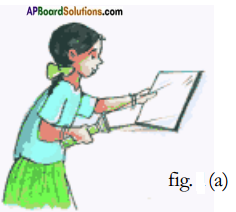2. Now turn the torch towards our face as shown in figure (b), and see our image in the mirror.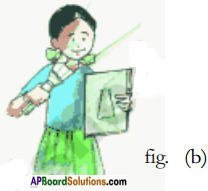3. We will find that when light is focused on our face we can see our image clearly in the mirror.
4. We also notice that when light is focused on the mirror we find a dim image of our face in the mirror.Question 2.
Explain by a simple activity what are incident and reflected rays of light. What is reflection of light?

1. Place a blank sheet of paper on the ground such that part of it is in the sunlight and other part of it is in the shadow.
2. Hold the mirror strip with the slit facing the sun.
3. Let a ray of light from the slit fall on the paper.
4. Now take another mirror strip and place it in the path of this light ray such that the ray coming from first mirror falls on the second mirror as shown in figure.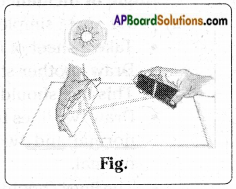5. We did not see any other ray of light, apart from the one from the mirror slit, on the paper.
6. This effect of the mirror strip on the ray of light is called reflection.
7. The ray of light falling on the mirror is called the incident ray and the ray returning from it is called the reflected ray.

Question 3.
Explain the terms ‘Angle of incidence’, ‘Angle of reflection’ and ‘Normal at the point of incidence.’

1. Take a sheet of blank paper. Draw a line segment AC across the middle.
2. Draw another straight fine at right angles (90 degrees) to segment AC.
3. The second line should bisect segment AC at point B. We shall call this line as Normal. See in figure, (a)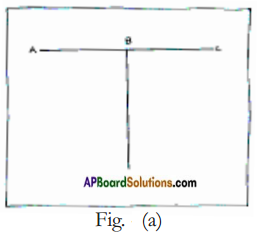4. Draw two lines from point B on the left side of the normal and two on the right side. The lines should be at angles of 30° and 60° respectively from the normal. Number these lines 1, 2, 3, 4 as shown in figure, (b)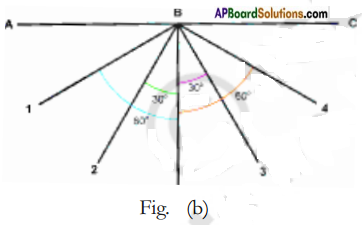5. Place a mirror strip vertically on segment AC with its reflecting surface facing the normal.
6. See that the back of the mirror coincides with segment with a slit and let its light ray fall along line 4.
7. Now this ray is the incident ray for the mirror.
8. The angle between the normal and the incident ray is called the Angle of Incidence (∠i).
9. The angle between normal and the reflected ray (along line 1) is called the Angle of Reflection (∠r).
10. Adjust the mirror strip with the slit so that its light ray falls along line 3 and we find that the reflected ray falls along line 2.
11. Adjust the mirror strip with the slit so that its light ray falls along normal, then the angle of incidence is 0° (the angle between normal and incident ray “that is also normal here”, is 0°)
12. The reflected light reflects back along the normal itself.Question 4.
What relationship exists between angle of incidence and angle of reflection? State it and prove the relation by a simple activity.

1. In the case of reflection on mirrors the law of reflection is Angle of incidence ∠i = Angle of reflection ∠r.
2. Proof : by a simple activity.
• Take a sheet of blank paper. Draw a line AC on it.
• Draw another straight line at right angles to AC.
• This line should bisect AC. This line is normal.
• Draw two lines from point B on the left side of the normal and two lines on the right side of the normal.
• The lines should make angles of 30° and 60° respectively from the normal. Number these lines as 1, 2, 3 and 4 as shown in figure.• Place the mirror strip vertically on AC. With its reflecting surface facing the normal.
• Take the mirror strip with a slit and let its light ray fall along line 4.
• We find the reflected ray coming along the line 1.
• Note the angle of incidence and angle of reflection.
• Repeat the experiment by sending the light ray along line 3.
• The reflected ray is found to be along line 2.
• Record the observations.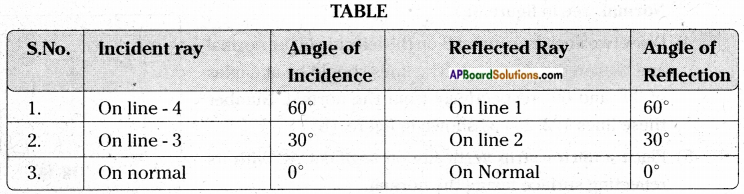It is clear from the above activity that angle of incidence ∠i = angle of reflection ∠r.

Question 5.
Describe a small activity to prove that it is possible to see the image of an object only when the incident ray, reflected ray and normal are at the same plane.

1. Place a mirror (1ft. × 1ft.) on the floor. You and your friends A, B, C stand on four sides of the mirror as shown in figure.
2. Adjust your places of standing, in such a way that each one of you can see the image of the person opposite to you in the mirror kept on the floor.
3. Your friends A, C are able to see images of each other in the mirror.
4. Ask your friend – B, who is in front of you to move a feet aside from his place. You cannot see his image in the mirror.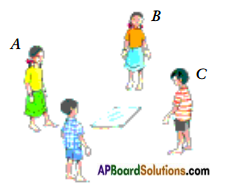5. Imagine a normal to the mirror. It would be perpendicular to the mirror as well as to the floor.
6. Imagine an incident ray coming from your friend B, falls on the mirror then the reflected ray from mirror reaches you.
7. Observe that the incident ray, reflected ray and normal lie in the same plane.
8. Now think why the image of your friend B is not visible to you when he moves aside. Where should.you stand to see his image? Once again imagine the incident, reflected rays and normal and assumed plane.
9. It is evident that the incident ray, reflected ray and normal should be in same plane, then only you can see your friend.Question 6.
How would you get an image in a mirror?

1. We see our image in a mirror many times. How it is formed?
2. During day time the light which falls on us gets reflected and a number of reflected light rays from us that fall on the mirror also get reflected back.
3. These reflected rays reach your eye and make us see our image.
4. See figure (a). Thus formation of image in mirrors is due to reflection of light rays from the mirror.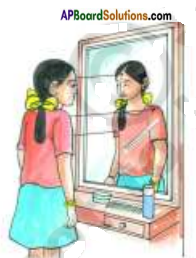5. See the figure (b) and observe the lines. They will explain how the image of a candle is formed in the mirror and how we are able to see the image of the candle in the mirror.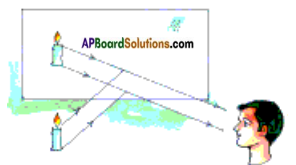Question 7.
What is the difference between you and your image in a mirror?

1. Stand in front of a mirror as shown in figure (a) and observe on which side of you does the pocket on your shirt appear, when you look into the mirror.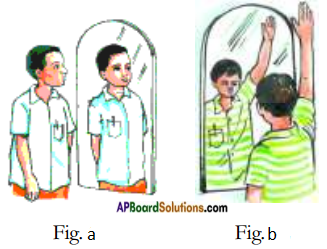2. Raise your right hand infront of a mirror as shown in figure (b). Which hand of your image appears raised?
3. To make your image to comb hair with its right hand, what will you have to do?
4. What do these observations suggest?
5. In a mirror, right of an object appears as left and left of the object appears as right.
6. Note that only sides are interchanged. This is called lateral inversion.Question 8.
How can you make a garden in a box ? Describe the procedure.

1. Take an empty shoe box. Place two plane mirrors along the edges.
2. See that the pair of mirrors are parallel to each other and their reflecting surfaces face each other as shown in figure.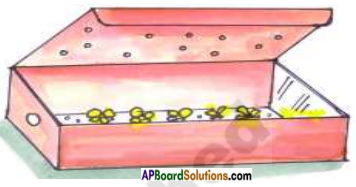3. Scratch the centre part of a mirror at its back and make a hole to the wall of the box such that it coincides with the scratched portion of the mirror.
4. Put some flowers in the box. Make a few holes in the lid before closing the box so that light enters into the box.
5. Now look through the hole. You can see a wonderful and beautiful vast garden.

Question 9.
Why does the small area look like a vast garden?

1. The light which enters the box falls on the flowers, gets reflected and travels in all directions.
2. The rays which fall on the mirrors reflect back to the opposite mirror.
3. This process happens again and again.
4. Due to this multiple reflection we can see that small area as a big garden.

Question 10.
Explain what do you understand by regular reflection and irregular reflection.

1. Reflection from a smooth surface like that of a mirror is called regular reflection (see figure a).
2. Clear images are formed in case of regular reflection.
3. Reflection from a rough or irregular surface is called irregular reflection or diffused reflection (see figure b).
4. Images are not clear in case of irregular reflection.
5. In some cases we can’t find the image at all.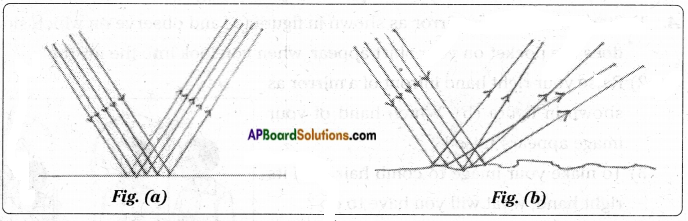Question 11.
Can we use rear view mirror in place of plain mirror? Yes /No give the reason.
No. We cannot use rear view mirror in place of plane mirror. The reasons are:

1. In plane mirror we can see our images.
2. But in rear view mirror we cannot see our images. So there is no use to use rear view mirror.

Question 12.
A mirror is hanging in your room. Your friend is sitting in the same room in a chair. If your friend wants to see you in a mirror, how will you adjust your position? Explain. ’
I will adjust my position according to the laws of reflection. According to the laws of reflection Angle of incidence = Angle of reflection. If my friend wants to see me in a mirror, I will adjust my position (i.e) the angle of incidence is equal to angle of reflection. Then my friend will see me in that mirror.

Question 13.
What are rays of light? How do you produce rays of light?

1. Take a mirror strip and a black paper as shown in figure, (a)
2. Cover the mirror strip with black paper and cut a 1 mm wide slit in the black paper, as shown in figure b.
3. Hold the mirror strip with the slit facing the sun as shown in figure, (c).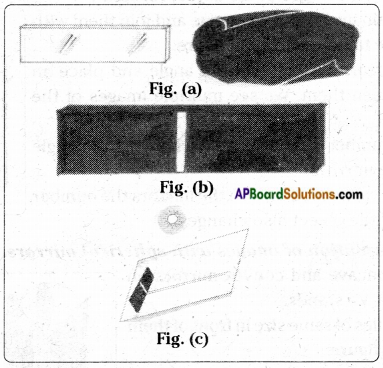4. We will see some light coming through the slit. Let this light fall on a sheet of paper spread on the ground.
5. Light coming from such a slit or any other small hole looks like a ray of light.

Question 14.
Why should we keep mirror strips parallel to each other in periscope? What happens if they are not parallel?

1. In a periscope the incident ray for the second mirror is the reflected ray of the first mirror.
2. When these two mirrors are parallel to each other, only then the rays lie in the same plane and can pass through the windows of the periscope.
3. If these mirrors make some angle with each other, then the reflected ray of the first mirror may not reach the second mirror or the reflected ray of second mirror may not pass through the window of periscope.
4. In both cases we can’t see the object with the periscope.Question 15.
Can you spell your name as it appears in a mirror? Spelling of my name is …………………..  It appears in the mirror as ……………
The student has to do it.

Question 16.
Show that the size of an object and its image are same.

1. Stand in front of a big mirror. Observe your image.
2. Step back two feet and step forward one foot. Observe your image while you move to and fro.
3. You notice that your image also move.
4. Estimate the distance from you to the mirror and the distance from the mirror to the image.
5. Is the size of your image equal to your size? Place an object in front of the mirror. Compare the size of the object with its image.
6. We notice that irrespective of the size of mirrors, the size of image and the size of object are equal.

Question 17.
How are multiple images of an object formed?

1. Take two plain mirrors of same size and join them with a cellophane tape as shown in figure.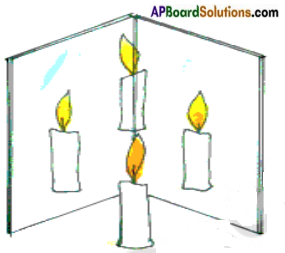2. Fold the mirrors through certain angle and place an object between them. We see multiple images of the object.
3. Count the number of images after changing the angle between the mirrors.
4. If we change the angle between the mirrors the number of images of the object also changes.

Question 18.
Explain the formation of images with spherical mirrors.

1. Place the concave and convex mirrors on two different V – stands.
2. Put two candles of same size in front of them as shown in figure.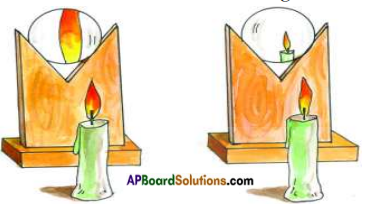3. Adjust the position of candles, to form clear images in the mirrors.
4. Observe the sizes of images.Question 19.
What are real and virtual images? Explain with the help of an activity.

1. Place a concave mirror on a V-stand.
2. Place a lighted candle in front of it. Place a thick white paper or white drawing sheet behind the candle.
3. This acts as a screen. See figure.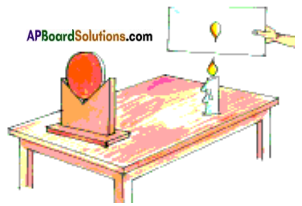4. Adjust distances between candle and mirror, screen and mirror by moving them either forward or backward till a clear image appear on the screen.
5. Repeat the activity using a convex mirror and plane mirror in place of concave mirror.
6. The image that can be obtained on a screen is called a Real Image. We can see this image in the mirror too.
7. The image that can’t be obtained on a screen but can be seen only in the mirror is
called a virtual image.

Question 20.
Explain how are we able to see the objects around us in day time?

1. We already know that, to see any object, light should fall on it.
2. In daytime we are able to see all objects which are in our room even though sunlight does not fall directly on those objects.
3. The multiple reflection of sunlight from surfaces like wall, floor, ceiling etc, finally falls on the object and gets reflected by it and reaches us.
4. This enables us to see the object.

Question 21.
How do you make a periscope?

1. We have to secure the following materials to make a periscope. Empty agarbatti box, two mirror strips, scale, pencil, blade, match box, candle, glue.
2. Close both ends of the agarbatti box. Draw squares at both ends.
3. Draw the diagonal to these squares as shown in figure.
4. Slit the diagonals with a blade. The slits should equal to the length of the mirror strips.
5. Fix the mirror strips in these slits as shown in figure (b). Take care to see that these mirror strips lie parallel to each other, with their reflecting surfaces facing each other.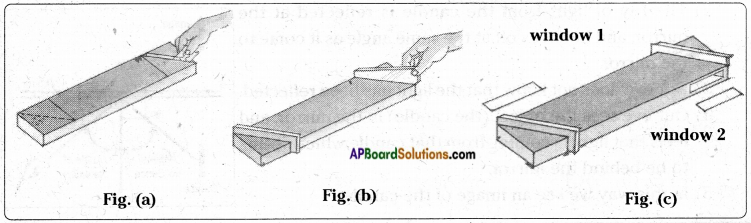6. Fix the mirror strips firmly to the box with a few drops of molten wax from a burning candle. We can also use glue or fevicol instead of wax.
7. Cut out two windows on the narrow sides of the box as shown in figure (c). The windows should open directly on the reflecting surfaces of the mirror strips.
8. Now the periscope is ready.Question 22.
How would you use the periscope?

1. When we look through window 2, We will be able to see things lying in front of window 1.
2. We hide behind a tree, we can easily see that is happening on the other side of the tree with this periscope.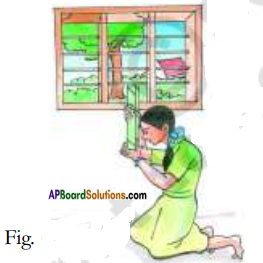3. Observe in figure the girl is viewing objects outside the room through the window while hiding herself in the room, with the help of a periscope.

Question 23.
Keep the mirrors in such a way that the angle between the mirrors is 90 degrees and observe the images and compare them with the object. What do you notice? Is there any difference between those images? Imagine the reason for that.

1. We observe 5 images of the object .
2. The intensity of the images decreases.

Question 24.
What are spherical mirrors?

1. We have seen the mirrors whose reflecting surfaces look like the head of a steel spoon.
2. The mirrors which contain curved reflecting surfaces are called spherical mirrors.

Question 25.
Why do we call concave and convex mirrors as spherical mirrors?

1. Take a rubber ball and cut a portion of it with knife as shown in figure. Be careful. Ask your teacher to help you in cutting the ball).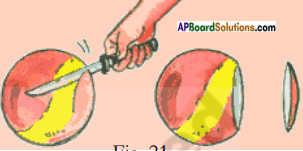2. The inner surface of the cut piece of ball is called concave surface and the outer surface of it is called convex surface.
3. If the reflecting surface of a mirror is concave, it is called a concave mirror see figure (a)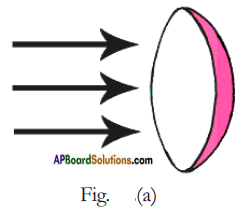4. If the reflecting surface is convex, then it is called as convex mirror. See figure (b).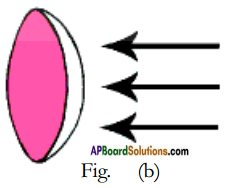5. Any concave or convex mirror is a part of a sphere. Hence these mirrors are called spherical mirrors.Question 26.
How is the image of a candle is seen in a mirror. Draw a diagram to explain.

1. See the diagram below.
2. The ray of light from the candle is reflected at the mirror, and bounces off at the same angle as it come to the mirror.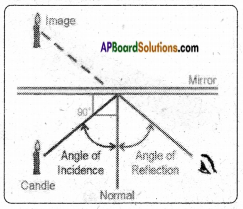3. Our eye does not know that the light has been reflected.
4. Our eye sees the object (the candle) in the mirror, and feels that light is coming from that candle which seems to be behind the mirror.
5. In this way we see an image of the candle.

Question 27.
Take a drawing sheet and cut it into a few pieces. Write an English letter in capitals on each piece of drawing sheet. Observe the images of the letters formed in the mirror as shown in figure.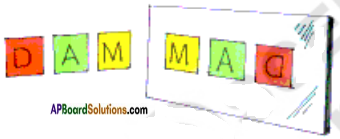1. Which of the images appear same as the original letter?
2. Which of them appear reversed? Why?

Repeat above activity with Telugu / Hindi / Urdu letters and also with numbers 1 to 9, then try to answer the above questions.
This can be done by the student.

Question 28.
On the front side of ambulances, the word AMBULANCE is written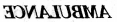like why?

1. All vehicles should give way to an Ambulance.
2. When the driver has seen the vehicle behind it, letters written on it must be clearly visible.
3. So the letters Ambulance are written on the vehicle with lateral inversion.
4. When the image of these letters are seen in the rear mirror, they will be laterally inverted and the name Ambulance will be clearly visible to him.
5. The driver immediately gives way to that Ambulance.

Question 29.
Every day we see our image in a plane mirror. Is it a real or virtual image? How can you decide?

1. It is a virtual image.
2. The image formed in a plane mirror cannot be caught on a screen.

Question 30.
Keep a mirror close to your face and look into it. Move the mirror backward and observe the image. What change do you observe? Try this activity using Convex, Concave mirrors. In which mirror do you get an inverted image ?At what distance does it happen?

1. When the mirror moves by a distance of minimum 25 cm. then only we can see our image in the plane mirror.
2. If it is a concave mirror, as long as our face it with in the focus of the concave mirror a small virtual image of the face is seen.
3. When the concave mirror is at a distance beyond its focus, then the image formed will be bigger in size.
4. In the case of convex mirror a virtual, small image is formed in it when it is kept at a suitable distance form the face.

Question 31.
Why are rearview mirrors arranged for vehicles?

1. The mirrors at both sides of driver in motor vehicles like bus, car, motor vehicles are rearview mirrors.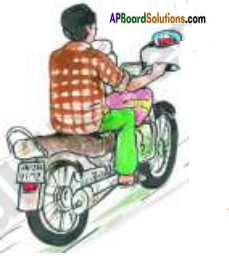2. These are used by drivers to see vehicles which are moving behind or beside of the vehicle without turningQuestion 32.
Take a plane mirror. Go to a vehicle. Observe your image in the rearview mirror and as well as in the plane mirror. What difference do you find?
We have noticed that the image formed by the rearview mirror is smaller than the image in a plane mirror.

Question 33.
a) How is the image on the outer portion of the spoon?
b) How is the image on the inner portion of the spoon?
a) The image on the outer portion of the spoon is small in size.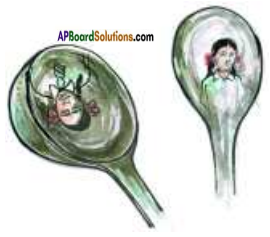b) The image on the inner portion of the spoon is reversed.

Question 34.
Have you noticed the surface of reflection in a torch light or in a head light of a vehicle? What is its specialty?

1. Observe the torch light or headlight of a vehicle.
2. We notice a concave mirror behind the bulb (see figure)
3. Due to this concave surface the brightness of a small bulb is increased.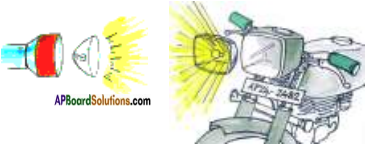Question 35.
What happens if the surface of reflection in the torch or in a head light of a vehicle is convex like in figure?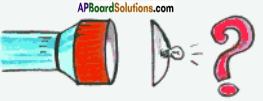1. The intensity of light totally decreases.
2. A convex mirror diverges the light rays.
3. So the rays of light from the bulb diverge out. Giving small intensity of light on the road.

Question 36.
How is concave mirror useful to dentists?

1. Dentists use concave mirrors to examine our teeth (See figure).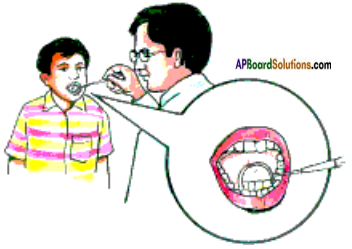2. These concave mirrors used by doctor help to see a bigger image of teeth.

Question 37.
In our daily life while we stand in front of windows we observe our images on the glass of some windows but don’t find images on the glass of some other windows. Why?

1. Our image is clear when we stand in front of certain types of glass as shown in figure (a).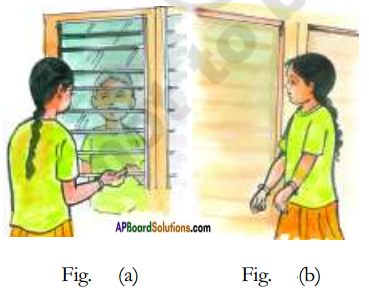2. Our image is not clear when we stand in front of some other types of glass as shown in figure (b).
3. If the surface of the window glass is smooth, due to the regular reflection we are able to see our image in that glass.
4. But if the surface of the window glass is rough, due to the irregular reflection we can’t find our image or we find unclear images in that glass.Question 38.
Observe the image of the sun or a tree in still water. Later, disturb the water by throwing a pebble. How does the image of the Sun or the tree appear now? Why?

1. When the water is still, its surface will be smooth and regular reflection takes place on it.
2. The image of an object formed due to still water will be clear.
3. When water is disturbed, the surface of water becomes rough and irregular reflection takes palce.
4. Due to this irregular reflection the image of an object may not be formed, if formed it will not be clear.

Question 39.
How many images are formed if two mirrors are kept in 60° angle to each other?
The angle between two plane mirrors = 60°
Number of images formed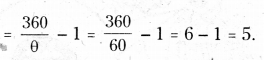5 images are formed.

Question 40.
Sasi asked her father to fix mirrors on her new house for elevation. But her father did not accepted. Imagine why he said like that.

1. If we decorate the front portion of the house, accidents may happen.
2. Because sunlight falls on these mirrors and reflects back.
3. This reflected light may fall on the men and vehicles, leading to accidents.

Question 41.
You are given a mirror. How can you identify whether it is convex mirror or concave mirror?
A)

1. If the reflecting surface of a mirror is concave, it is called a concave mirror.
2. These mirrors form real and inverted images.
3. These are used in headlights of vehicles. ENT doctors also use these mirrors for their observations.

B)

1. If the reflecting surface is convex, then it is called a convex mirror.
2. They always form erect and virtual images.
3. These are used in rear view mirrors of the vehicles.

Question 42.
The following experiment has been conducted in your laboratory.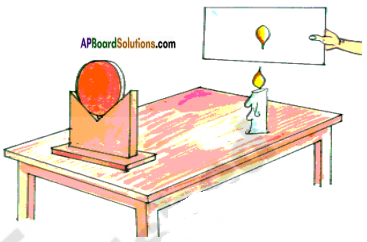Answer the following questions based on this.

1. Which mirror is used in this experiment?
2. How does the image of candle appear on the screen?
3. Since the image is caught on the screen, what is the name of the image?
4. What are the apparatus required to conduct this experiment?

1. Concave mirror
2. Inverted
3. Real image
4. U-stand, mirrors, candle, matchbox, screen, scale.Question 43.
List out the properties of a convex mirror.